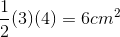# GMAT Math : DSQ: Calculating the area of a right triangle

## Example Questions

### Example Question #1 : Dsq: Calculating The Area Of A Right Triangle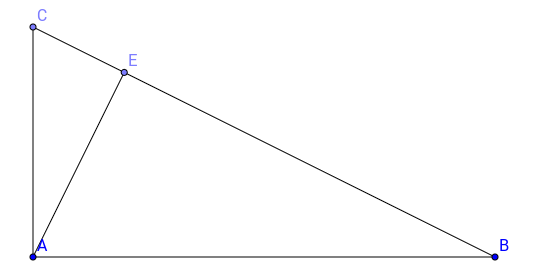Given: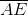is the height of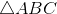.

What is the area of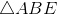?

(1)is a right triangle where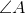is a right angle.

(2)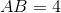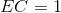Statement (1) is sufficient

Both statements are sufficient

Each statement alone is sufficient

Statement (2) is sufficient

Statement (1) and (2) taken together are not sufficient

Statement (1) and (2) taken together are not sufficient

Explanation:

Here, we would need to know the length of at least two sides of the triangle ABC, provided it is a right triangle, in order to calculate the area of ABE.

Statement 1 is insufficient alone because it only tells us a property of the triangle and gives us no information about the lengths of the sides. Similarily, statement 2 alone is insufficient because we can't tell the area of triangle ABE from what is given. We would need the length of the height and the length of EB.

Statements 1 and 2 taken together are insufficient, because even though the height divides the triangle in two similar triangles, we can't find any ratio to calculate the length of the height AE. Therefore, Statements 1 and 2 taken together are insufficient.

### Example Question #2 : Dsq: Calculating The Area Of A Right Triangle

Find the area of the right triangle.

Statement 1):   The hypotenuse is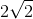.

Statement 2):   Both legs have a side length of.

BOTH statements TOGETHER are NOT sufficient, and additional data is needed to answer the question.

BOTH statements taken TOGETHER are sufficient to answer the question, but neither statement ALONE is sufficient.

EACH statement ALONE is sufficient.

Statement 1) ALONE is sufficient, but Statement 2) ALONE is not sufficient to answer the question.

Statement 2) ALONE is sufficient, but Statement 1) ALONE is not sufficient to answer the question.

Statement 2) ALONE is sufficient, but Statement 1) ALONE is not sufficient to answer the question.

Explanation:

Statement 1) only provides the hypotenuse of the triangle, but it does not imply that both legs of the right triangle are congruent sides.  Of the three interior angles, only the right angle is known with the other 2 unknown interior angles.

Statement 1) does not have enough information to solve for the area of the triangle.

Statement 2) provides the lengths of both legs.  The formula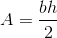can be used to solve for the area of the triangle.

Statement 2) can be used by itself to solve for the area of the triangle.

### Example Question #3 : Dsq: Calculating The Area Of A Right Triangle

The lobby of a building is in the shape of a right triangle. The shortest side of the room ismeters long. Find the number of tiles needed to cover the floor.

I) Each tile coverssquare centimeters.

II) The longest side of the lobby is five less than three times the shortest side.

Both statements are needed to answer the question.

Either statement is sufficient to answer the question.

Statement II is sufficient to answer the question, but statement I is not sufficient to answer the question.

Statement I is sufficient to answer the question, but statement II is not sufficient to answer the question.

Both statements are needed to answer the question.

Explanation:

To find the number of tiles needed, we need to find the area of the lobby and the area of one tile.

I) Gives us the area of one tile.

II) Gives us the length of the hypotenuse of the lobby.

Use II) along with the info given in the question to find the last side (Pythagorean Theorem).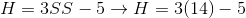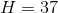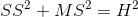, where SS is the short side, MS is the middle side, and H is the hypotenuse.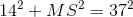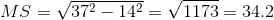From there you can find the area of the lobby.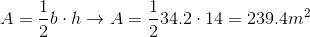Use I) along with the area of the lobby to find the number of tiles needed.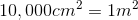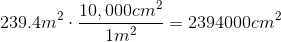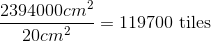### Example Question #4 : Dsq: Calculating The Area Of A Right Triangle

What is the area of the right triangle?

1. The hypotenuse measures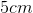.
2. The height measures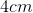.

Statements 1 and 2 are not sufficient, and additional data is needed to answer the question.

Statement 1 alone is sufficient, but statement 2 alone is not sufficient to answer the question.

Each statement alone is sufficient to answer the question.

Both statements taken together are sufficient to answer the question, but neither statement alone is sufficient.

Statement 2 alone is sufficient, but statement 1 alone is not sufficient to answer the question.

Both statements taken together are sufficient to answer the question, but neither statement alone is sufficient.

Explanation:

Neither statement provides us with sufficient information to answer the question but both statements taken together are sufficient to answer the question.

In order to find the area of the right triangle we need both the height and base. We can use the Pythagorean Theorem to solve for the base.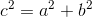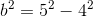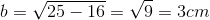Now we can find the area: